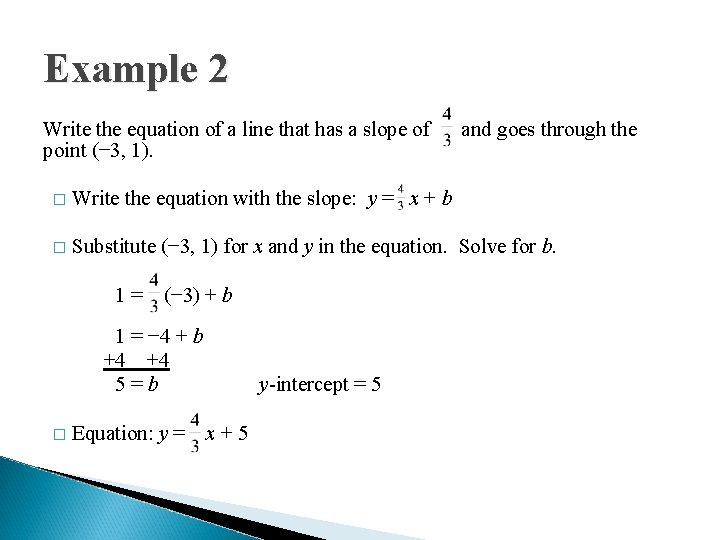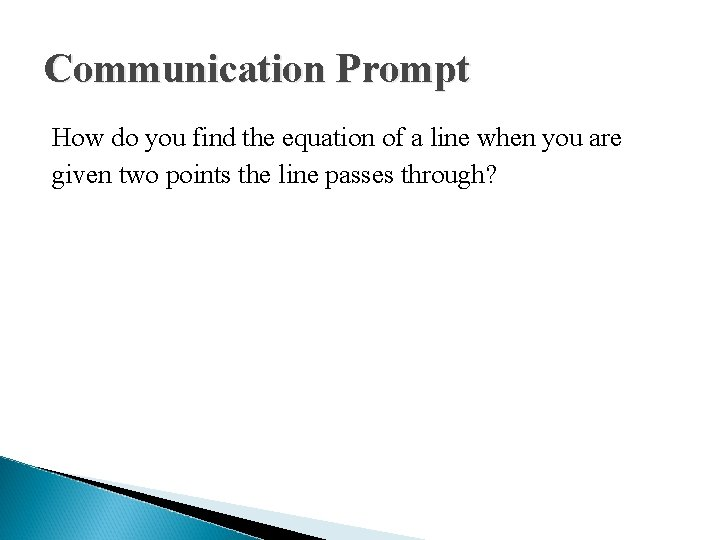# Lesson 18 Linear Equations Writing Linear Equations From

• Slides: 9Lesson 18 Linear Equations Writing Linear Equations From Key InformationWarm-Up Graph each linear equation. Clearly mark at least three points on each line. 1. 2. y = − 2 xWriting Linear Equations From Key Information Target: Write a linear equation in slope-intercept form when given information about the line.Example 1 Write the equation of a line that has a slope of − 2 and a y-intercept of 5. � General � Slope: Equation: y = mx + b m = − 2 � y-intercept: � Equation: b=5 y = − 2 x + 5Writing a Linear Equation when Given Key Information 1. Find the slope (m) of the line. 2. Find the y-intercept (b) of the line. If necessary, substitute the slope for m and one ordered pair (x, y) for the corresponding variables in the equation y = mx + b. Solve for b. 3. Write the equation in the form y = mx + b.Example 2 Write the equation of a line that has a slope of point (− 3, 1). and goes through the � Write the equation with the slope: y = x + b � Substitute (− 3, 1) for x and y in the equation. Solve for b. 1= (− 3) + b 1 = − 4 + b +4 +4 5=b � Equation: y = y-intercept = 5 x+5Exit Problems Write the equation of each line in slope-intercept form. 1. slope = 4 y-intercept = − 5 2. slope = 3. goes through (0, 4) and (− 2, 8) goes through (2, 6)Communication Prompt How do you find the equation of a line when you are given two points the line passes through?